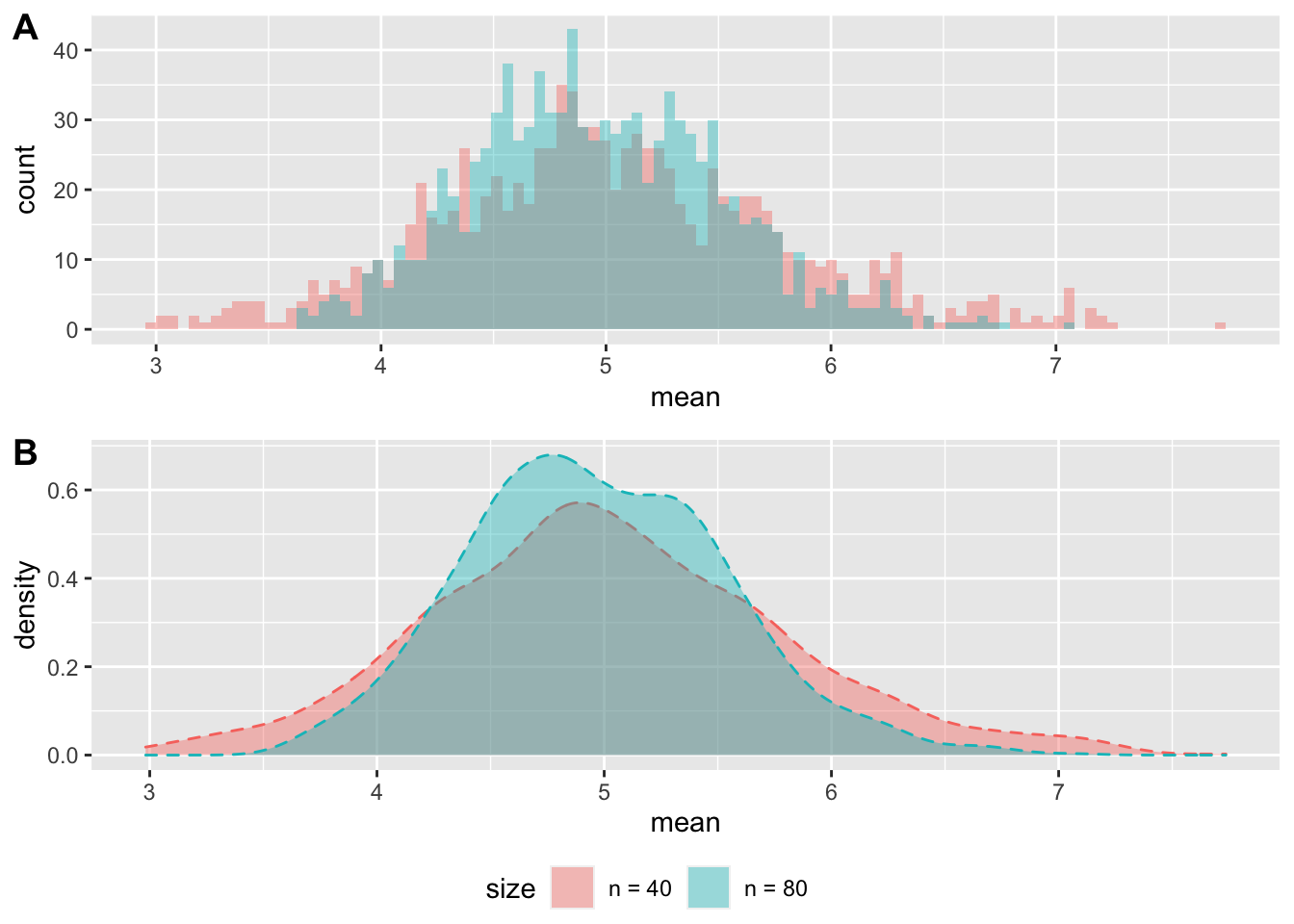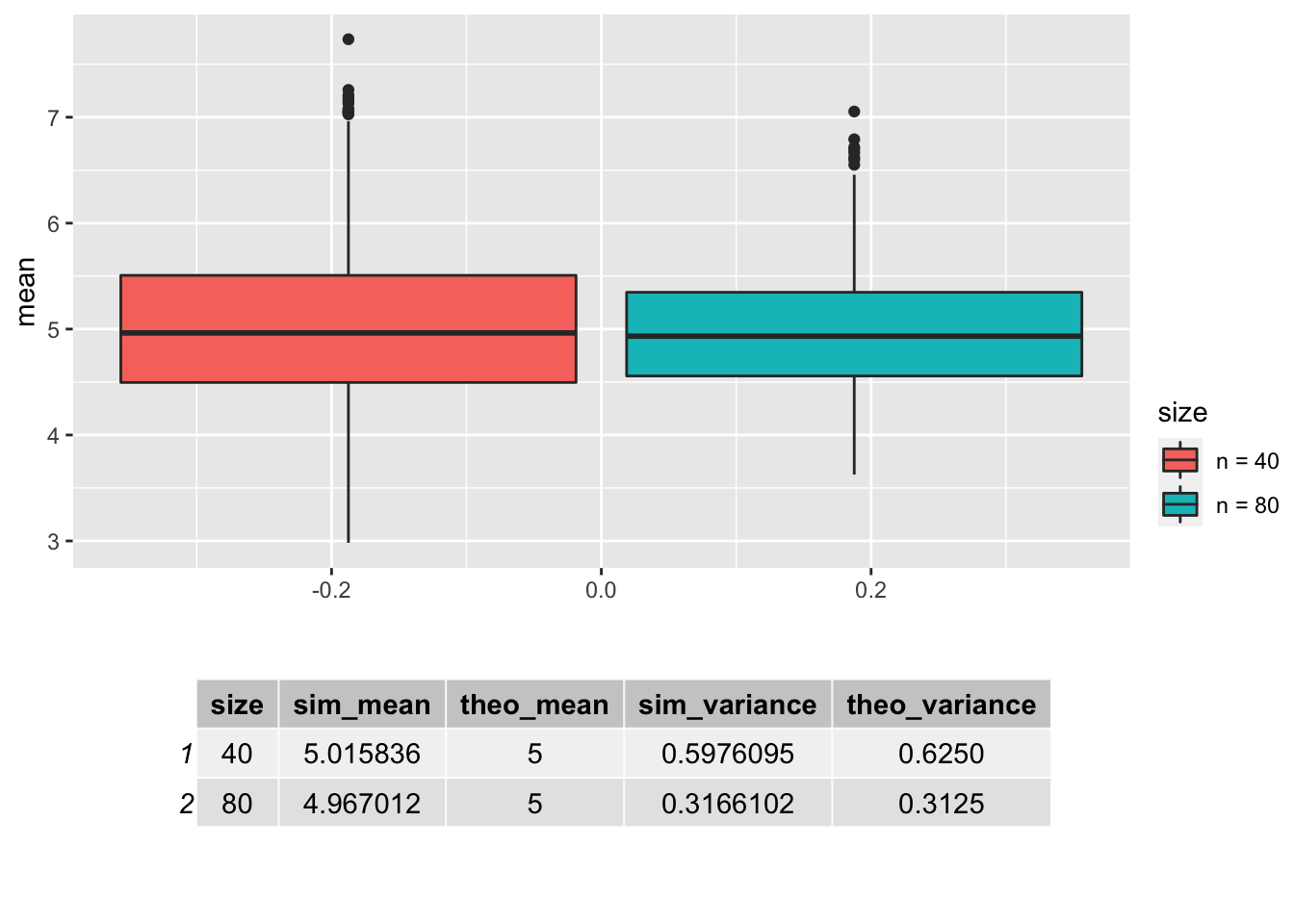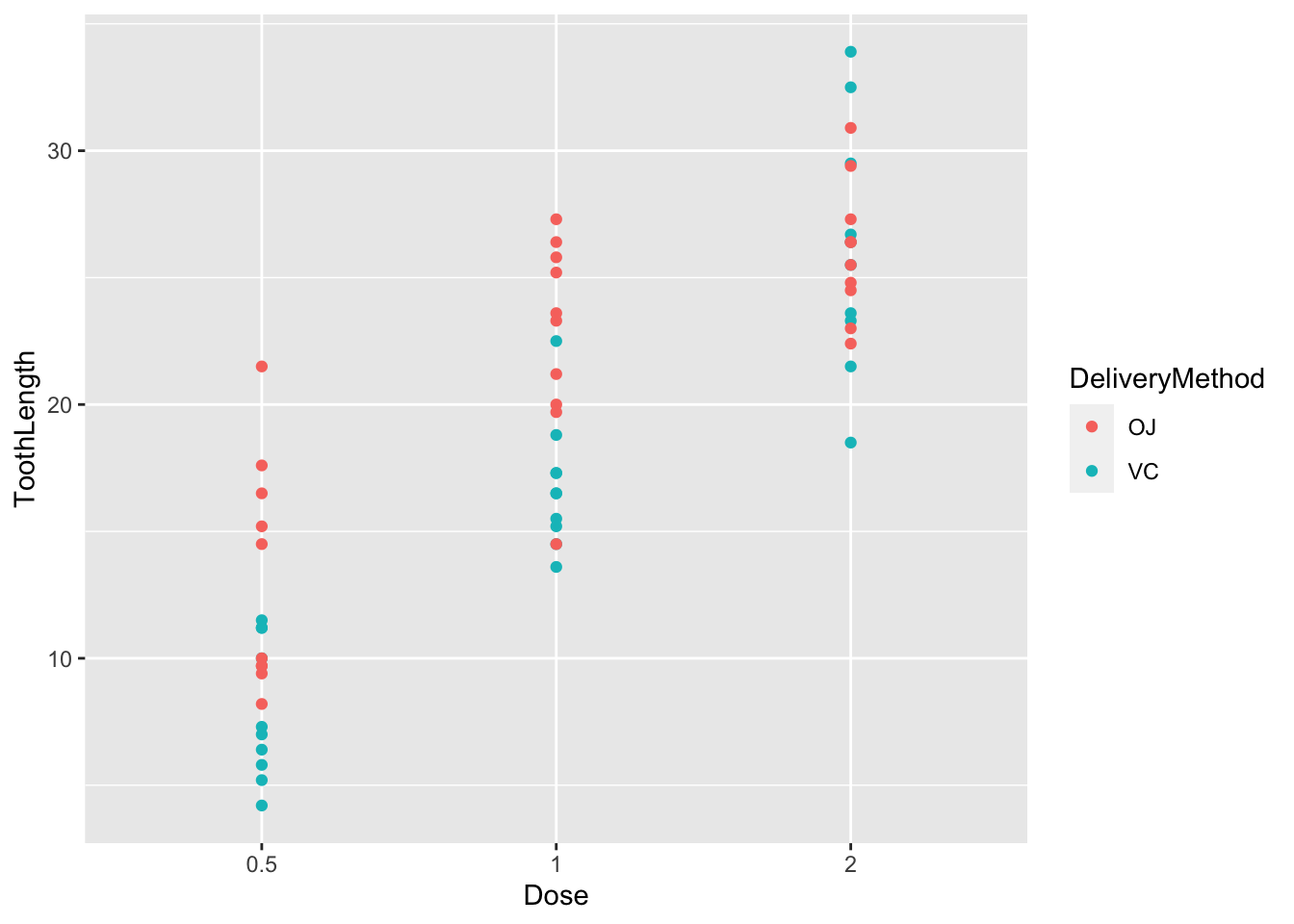# Course Project

## Part one: Simulation Exercise

The exponential distribution is one of the most popular and useful distributions in statistical analysis. It is used to model the number of events2 occurring over a period of time. Let $$X$$ be the number of events over the observation period $$t$$: $$X \sim {\sf Exp}(\lambda t)$$ with $$\lambda$$ representing the expected number of events per time unit (minute, hour, etc.).$$\lambda$$ is often referred to as the rate parameter. The probability density function (PDF) is given by $$f(x;\lambda) = \lambda e^{-\lambda x}$$ for $$x \geq 0$$ and $$f(x;\lambda) = 0$$ for $$x <0$$. By integrating $$f(x,\lambda)$$ one receives $$F(x;\lambda) = 1-e^{-\lambda x}$$, i. e. the cumulative distribution function (CDF). Interestingly, the mean and the standard deviation of X are both $$\frac{1}{\lambda}$$, with the variance being $$Var(X) = \frac{1}{\lambda^2}$$.

The goal of this study lies in observing the behavior of the exponential distribution, especially regarding its mean. Using R, it is possible to compare theoretical findings to a simulation. Let the rate parameter be $$\lambda = 0.2$$ and the sample size $$n$$ be equal to 20. It is known, that the expected value of the distribution is $$E[X] = \frac{1}{\lambda}$$, one would therefore expect a sample mean of $$\bar{X} = \frac{1}{0.2} = 5$$.

set.seed(101)
n <- 40
lambda <- 0.2
mean(rexp(n,rate = lambda))
##  4.034012

We see that the sample mean is close to its theoretical counterpart. The law of large numbers would predict, that the sample mean limits to the theoretical mean as the sample size grows.

n <- 400
mean(rexp(n,rate = lambda))
##  4.818306

And indeed, the distance between the theoretical and sample mean did shrink markedly by increasing the sample size by a factor of 10. This finding, as intuitive as it might be, poses a theoretical question about the distribution of sample means. Using simple algebra to receive the sample (arithmetic3-) means expected value and variance, one arrives at $$E[\bar{X}] = \mu$$ and $$E[Var(\bar{X})] = \frac{\sigma^2}{n}$$. It is essential to realize that statistics, such as the sample mean, are calculated based on realizations drawn randomly from a population, are themselves random variables. The first moment, $$E[\bar{X}]$$ of the distribution of the sample means implies a centering around the expected value of the underlying distribution. This can be tested by simulating 1.000 samples of size 40 and 80 from the exponential function characterized above and taking the mean of each. The resulting distributions are depicted as a histogram (A) and as a density (B).

mns_40 = NULL
mns_80 = NULL
for (i in 1 : 1000) mns_40 = c(mns_40, mean(rexp(40, rate = lambda)))
for (i in 1 : 1000) mns_80 = c(mns_80, mean(rexp(80, rate = lambda)))
mns <- data.frame(mean = c(mns_40,mns_80),size = rep(c("n = 40", "n = 80"),each =1000))
library(ggplot2)
library(ggpubr)
g = ggplot(mns, aes(x=mean, fill=size))
g = g + geom_histogram(alpha=0.4, position="identity", bins = 100)
d = ggplot(mns, aes(x=mean, fill=size))
d = d + geom_density(alpha = 0.4, aes(color = size),linetype = 2)
ggarrange(g,d, nrow = 2, align = "hv",common.legend = TRUE,
legend = "bottom", labels = c("A","B"))By visually examining the mean distributions, it is possible to make 3 distinct observations:

1. both distributions are centered around the theoretical mean,
2. increasing the sample size leads to a less flat distribution, i. e. lower variance,
3. the distributions of $$\bar{X}$$ appear quite Gaussian since they take a bell curve shape.

Findings 1-3 are in line with the central limit theorem (CLT) which would predict such a behavior of sample means. This enables the statistician to apply techniques for normal distributions to sample means and other select statistics and make useful inferences about the underlying data-creating processes.

sim_variance <- c(var(mns_40), var(mns_80))
sim_mean <- c(mean(mns_40), mean(mns_80))
theo_variance <- c((1/0.04)/40, (1/0.04)/80)
theo_mean <- c(1/lambda, 1/lambda)
df <- data.frame(size = c("40","80"),sim_mean, theo_mean, sim_variance, theo_variance)
p = ggplot(mns, aes(x =mean, fill=size)) + geom_boxplot(aes(fill = size)) + coord_flip()
t = ggtexttable(df)
ggarrange(p,t, nrow = 2, align = "hv",common.legend = TRUE,
legend = "right", heights = c(2,1), widths = c(1,2) )A comparison between the realized first moments of the simulated distribution of means to their theoretical counterparts, as suggested by the CLT, is conducted (see above). Indeed, the simulated moments are very close to what the theory would suggest, indicating that the CLT holds true.

## Part 2: Basic Inferential Data Analysis

To illustrate the application of inferential statistics a brief analysis of the “ToothGrowth” data set is performed. The data is based on an experiment4 where the effect of vitamin c on teeth growth in guinea pigs was studied. The data comprises 60 observations which are split into two groups of 30 observations each, categorized by the delivery method of the vitamin. The first group received their doses via orange juice (OJ), the second via ascorbic acid (coded VC). The researchers varied the doses given (0.5, 1, and 2 mg/day), this originally numerical column is converted to a categorical.

TG =  ToothGrowth
TG[,3] = as.factor(TG[,3])
colnames(TG) = c("ToothLength", "DeliveryMethod", "Dose")
summary(TG)
##   ToothLength    DeliveryMethod  Dose
##  Min.   : 4.20   OJ:30          0.5:20
##  1st Qu.:13.07   VC:30          1  :20
##  Median :19.25                  2  :20
##  Mean   :18.81
##  3rd Qu.:25.27
##  Max.   :33.90
ggplot(TG, aes(x=Dose, y = ToothLength)) + geom_point(aes(color = DeliveryMethod))By visually examining the data in the plot above, it is easy to observe a positive relationship between teeth growth and the dosage of vitamin c. The guinea pigs which received their dosage via orange juice (OJ) also seem to have experienced stronger growth as those which received ascorbic acid (VC). We can test these findings by applying standard t-tests to the mean teeth growth between the two groups of delivery methods and the low and the high dosage level (0.5 mg vs. 2 mg).

# Testing for differences between the delivery methods.
OJ = TG$ToothLength[TG$DeliveryMethod == "OJ"]
VC = TG$ToothLength[TG$DeliveryMethod == "VC"]
pvalues_Delivery = c(t.test(OJ,VC, alternative = "two.sided")$p.value, t.test(OJ,VC, alternative = "greater")$p.value)
# Testing for differences between the low and the high dosage (LD, HD).
LD <- TG$ToothLength[TG$Dose == "0.5"]
HD <- TG$ToothLength[TG$Dose == "2"]
pvalues_Dose = c(t.test(HD,LD, alternative = "two.sided")$p.value, t.test(HD,LD, alternative = "greater")$p.value)
# Compiling the results
pvals <- data.frame(pvalues_Dose, pvalues_Delivery,
row.names = c("two-sided", "greater"))
colnames(pvals) <- c("H0: High Dosage of Vitamin C has no effect on teeth growth.",
"H0: Delivery Method has no effect on teeth growth.")
pvals

It is possible to reject the hypothesis, that the higher dosage of vitamin c is not associated with increased teeth growth, in favor of the alternative in both the one-sided as well as the two-sided test at an confidence level of less than 1%. This strongly indicates, that vitamin c does have an positive effect on teeth growth. The effect of the different delivery method does remain ambiguous as we cannot reject the null-hypothesis of the two-sided test at a statistical significance of 5%. These findings are based on the assumption, that the data is independent and identically distributed (i.i.d) and that the central limit theorem holds true.

1. Humboldt University of Berlin↩︎

2. E. g. the failure of an electric or mechanical component, the death of a patient in a hospital or the outbreak of measles in a daycare.↩︎

3. $$\bar{X} = \frac{1}{n}\sum_{i = 1}^{n}x_i$$↩︎

4. Crampton, E. W. (1947). The growth of the odontoblast of the incisor teeth as a criterion of vitamin C intake of the guinea pig. The Journal of Nutrition, 33(5), 491–504. doi:10.1093/jn/33.5.491.↩︎